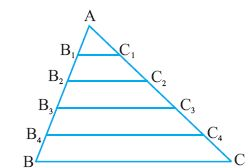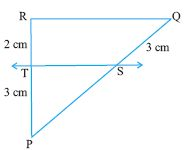Name: ___________________Date:___________________

 Email us to get an instant 20% discount on highly effective K-12 Math & English kwizNET Programs!

High School Mathematics - 28.1 Basic Proportionality Theorem or Thale's Theorem

A straight line drawn parallel to a side of a triangle, divides the other two sides proportionally.
Data: Triangle ABC, D is a point on AB and the line DE is parallel to BC
To prove: AD/BD = BE/CEConsider triangle ABC and triangle BDE
 Step Statement Reason 1 ang BAC = ang BDE Corresponding angles because DE ll AC 2 ang BCA = ang BED Corresponding angles because DE ll AC 3 ang DBE = ang ABC Common angle 4 triangle ABC lll triangle BDE AAA postulate on similarity 5 AB/BD = BC/BE corresponding sides are proportional 6 (BD+DA)/BD =(BE+EC)/BE Split AB and AC 7 1+AD/BD = 1+CE/BE Simplification 8 AD/BD = CE/BE Subtract 1 from either sides 10 AD/BD= BE/CE Reciprocating

Converse
We shall try to prove the converse of basic proportionality theorem.
Let us consider triangle ABC shown in the figure below.Divide the sides of the triangle AB and AC into any number of equal parts.
By measuring the angles B1 and B we observe that they are equal.
But B1 and B are corresponding angles.
Hence B1C1 ll BC.
Similarly
AB2/B2 B= AC2/C2C = 2/3 and B22C2 ll BC
AB3/B3B =AC3/C3C= 3/2 and B3C3 ll BC
AB4/B4B = AC4/C4C and B4C4 ll BC

Example: In the figure, find the length of PS given that ST ll QR.Let PS = x cm
As ST ll QR
PS/QS = RT/PT
x/3 = 3/2
x = 9/2
Answer: PS = 4.5 cms

Directions: Answer the following questions.
 Q 1: In the figure, is PQ ll EF. State yes or no.noyes Q 2: In the figure, are the lines EF, AB and CD parallel to each other. State yes or no.noyes Q 3: M and N are points on the sides PQ and QR of triangle PQR. If PM is 4, QM = 4.5, NR = 4.5 cm. find the length of PN4.5 cm4 cm Q 4: The diagonals AC and BD of a quadrilateral ABCD intersect at O such that AO/OC = BO/OD. In such a case, ABCD becomes a trapezium.falsetrue Q 5: In a trapezium ABCD, AB ll CD. Its diagonals AC and BD intresect at O. Then AO.OD = BO.OCtruefalse Q 6: ABC is a triangle in which D and E are the points on AC and BC. DE ll AB. CD = 15 cms, AB = 11 cms, DE = 7cms. Find the length of CD9.38.57 Q 7: ABC is a triangle. D and E are points on AB and AC. If BD = 4 cms, AD = 8 cms, CE = 9 cms. Find BE.3.5 cms4.5 cms2.5 cms Q 8: In triangle ABC, D and E are the points on AB and AC. BD = 30, DA = x, BF = y, EC = 15, AC = 22. Find AD.17.11510 Question 9: This question is available to subscribers only! Question 10: This question is available to subscribers only!

Subscription to kwizNET Learning System costs less than \$1 per month & offers the following benefits:

• Unrestricted access to grade appropriate lessons, quizzes, & printable worksheets
• Instant scoring of online quizzes
• Progress tracking and award certificates to keep your student motivated
• Unlimited practice with auto-generated 'WIZ MATH' quizzes